# 机器学习系列(9)_机器学习算法一览（附Python和R代码）

http://blog.csdn.net/longxinchen_ml/article/details/51192086

– 埃里克 施密特（谷歌首席执行官）

## 常见的机器学习算法

1.线性回归 (Linear Regression)

2.逻辑回归 (Logistic Regression)

3.决策树 (Decision Tree)

4.支持向量机（SVM）

5.朴素贝叶斯 (Naive Bayes)

6.K邻近算法（KNN）

7.K-均值算法（K-means）

8.随机森林 (Random Forest)

9.降低维度算法（Dimensionality Reduction Algorithms）



### 1.线性回归 (Linear Regression)

• Y- 因变量

• a- 斜率

• X- 自变量

• b- 截距

a和b可以通过最小化因变量误差的平方和得到（最小二乘法）。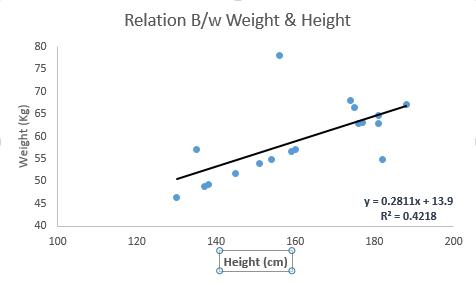Python 代码

#Import Library
#Import other necessary libraries like pandas, numpy...
from sklearn import linear_model
#Identify feature and response variable(s) and values must be numeric and numpy arrays

x_train=input_variables_values_training_datasets
y_train=target_variables_values_training_datasets
x_test=input_variables_values_test_datasets

# Create linear regression object
linear = linear_model.LinearRegression()

# Train the model using the training sets and check score
linear.fit(x_train, y_train)
linear.score(x_train, y_train)

#Equation coefficient and Intercept
print('Coefficient: \n', linear.coef_)
print('Intercept: \n', linear.intercept_)

#Predict Output
predicted= linear.predict(x_test)


R 代码

#Load Train and Test datasets
#Identify feature and response variable(s) and values must be numeric and numpy arrays

x_train <- input_variables_values_training_datasets
y_train <- target_variables_values_training_datasets
x_test <- input_variables_values_test_datasets
x <- cbind(x_train,y_train)

# Train the model using the training sets and check score
linear <- lm(y_train ~ ., data = x)
summary(linear)

#Predict Output
predicted= predict(linear,x_test)


### 2.逻辑回归

odds= p/ (1-p) = probability of event occurrence / probability of not event occurrence

ln(odds) = ln(p/(1-p))

logit(p) = ln(p/(1-p)) = b0+b1X1+b2X2+b3X3....+bkXkPython 代码

#Import Library
from sklearn.linear_model import LogisticRegression
#Assumed you have, X (predictor) and Y (target) for training data set and x_test(predictor) of test_dataset

# Create logistic regression object

model = LogisticRegression()

# Train the model using the training sets and check score
model.fit(X, y)
model.score(X, y)

#Equation coefficient and Intercept
print('Coefficient: \n', model.coef_)
print('Intercept: \n', model.intercept_)

#Predict Output
predicted= model.predict(x_test)


R 代码

x <- cbind(x_train,y_train)

# Train the model using the training sets and check score
logistic <- glm(y_train ~ ., data = x,family='binomial')
summary(logistic)

#Predict Output
predicted= predict(logistic,x_test)

• 加入交互项（interaction）

• 减少特征变量

• 正则化（regularization

• 使用非线性模型

### 3.决策树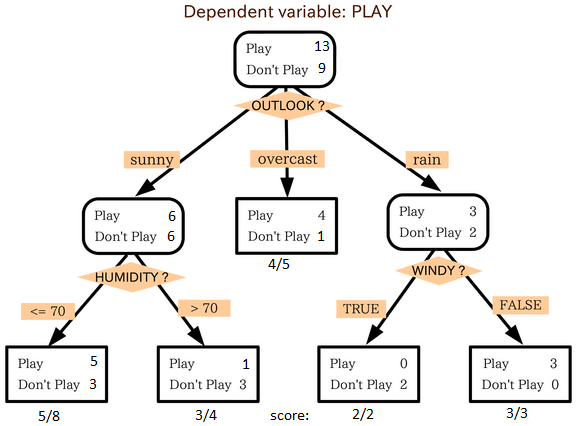Python 代码


#Import Library
#Import other necessary libraries like pandas, numpy...

from sklearn import tree
#Assumed you have, X (predictor) and Y (target) for training data set and x_test(predictor) of test_dataset

# Create tree object
model = tree.DecisionTreeClassifier(criterion='gini') # for classification, here you can change the algorithm as gini or entropy (information gain) by default it is gini

# model = tree.DecisionTreeRegressor() for regression

# Train the model using the training sets and check score
model.fit(X, y)
model.score(X, y)

#Predict Output
predicted= model.predict(x_test)

R 代码

library(rpart)
x <- cbind(x_train,y_train)

# grow tree
fit <- rpart(y_train ~ ., data = x,method="class")
summary(fit)

#Predict Output
predicted= predict(fit,x_test)

### 4. 支持向量机（SVM）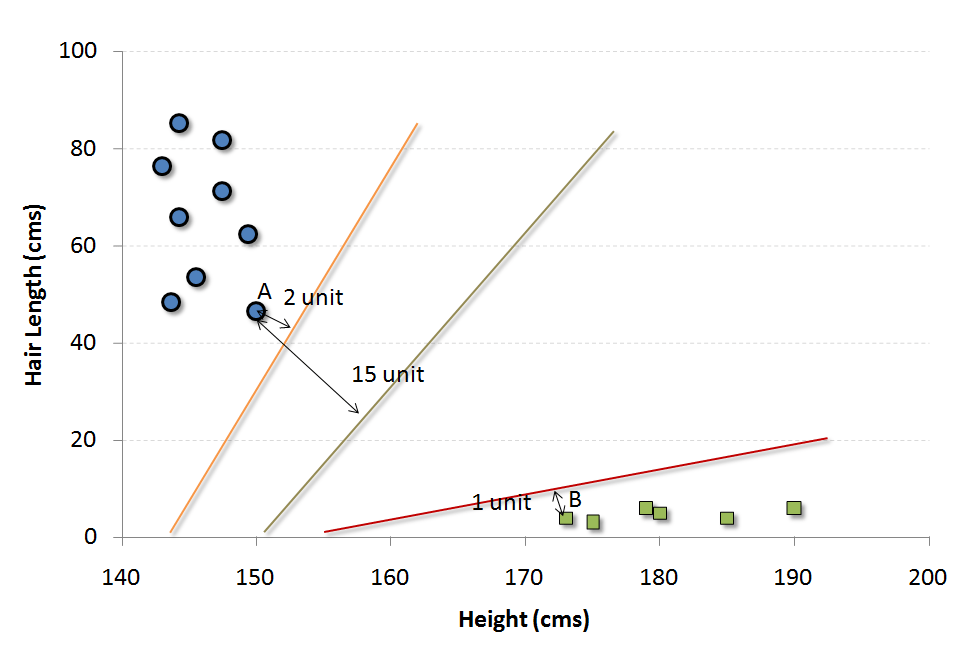#### 我们可以把这个算法想成n维空间里的JezzBall游戏，不过有一些变动：

• 你可以以任何角度画分割线/分割面（经典游戏中只有垂直和水平方向）。

• 现在这个游戏的目的是把不同颜色的小球分到不同空间里。

• 小球是不动的。

Python 代码


#Import Library
from sklearn import svm
#Assumed you have, X (predictor) and Y (target) for training data set and x_test(predictor) of test_dataset
# Create SVM classification object

model = svm.svc() # there is various option associated with it, this is simple for classification. You can refer link, for mo# re detail.

# Train the model using the training sets and check score
model.fit(X, y)
model.score(X, y)

#Predict Output
predicted= model.predict(x_test)

R 代码

library(e1071)
x <- cbind(x_train,y_train)

# Fitting model
fit <-svm(y_train ~ ., data = x)
summary(fit)

#Predict Output
predicted= predict(fit,x_test)


### 5. 朴素贝叶斯• P(c|x)是已知特征x而分类为c的后验概率。

• P(c)是种类c的先验概率。

• P(x|c)是种类c具有特征x的可能性。

• P(x)是特征x的先验概率。Python 代码

#Import Library
from sklearn.naive_bayes import GaussianNB
#Assumed you have, X (predictor) and Y (target) for training data set and x_test(predictor) of test_dataset

# Create SVM classification object model = GaussianNB() # there is other distribution for multinomial classes like Bernoulli Naive Bayes, Refer link

# Train the model using the training sets and check score
model.fit(X, y)

#Predict Output
predicted= model.predict(x_test)

R 代码

library(e1071)
x <- cbind(x_train,y_train)
# Fitting model

fit <-naiveBayes(y_train ~ ., data = x)
summary(fit)

#Predict Output
predicted= predict(fit,x_test)

### 6.KNN（K-邻近算法）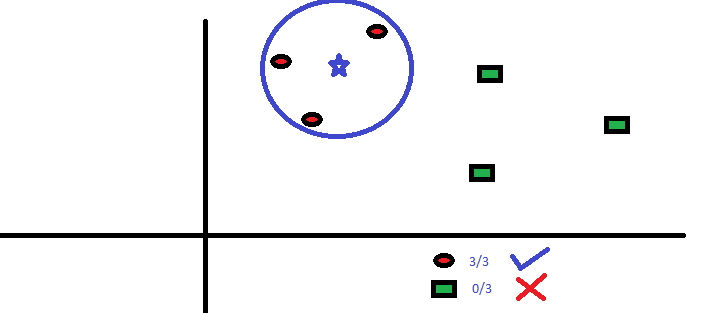KNN在生活中的运用很多。比如，如果你想了解一个不认识的人，你可能就会从这个人的好朋友和圈子中了解他的信息。

• KNN的计算成本很高

• 所有特征应该标准化数量级，否则数量级大的特征在计算距离上会有偏移。

• 在进行KNN前预处理数据，例如去除异常值，噪音等。

Python 代码

#Import Library
from sklearn.neighbors import KNeighborsClassifier

#Assumed you have, X (predictor) and Y (target) for training data set and x_test(predictor) of test_dataset
# Create KNeighbors classifier object model

KNeighborsClassifier(n_neighbors=6) # default value for n_neighbors is 5

# Train the model using the training sets and check score
model.fit(X, y)

#Predict Output
predicted= model.predict(x_test)

R 代码

library(knn)
x <- cbind(x_train,y_train)

# Fitting model
fit <-knn(y_train ~ ., data = x,k=5)
summary(fit)

#Predict Output
predicted= predict(fit,x_test)

### 7. K均值算法（K-Means）K均值算法如何划分集群：

1. 从每个集群中选取K个数据点作为质心（centroids）。

2. 将每一个数据点与距离自己最近的质心划分在同一集群，即生成K个新集群。

3. 找出新集群的质心，这样就有了新的质心。

4. 重复2和3，直到结果收敛，即不再有新的质心出现。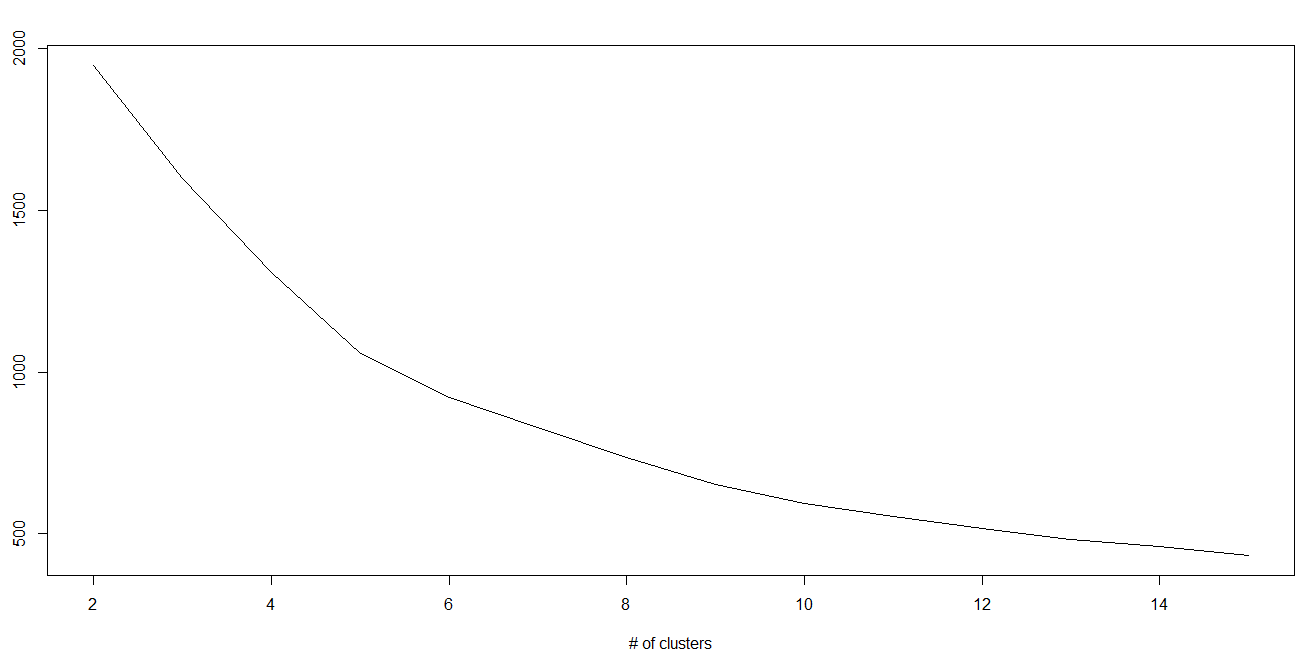Python 代码

#Import Library
from sklearn.cluster import KMeans

#Assumed you have, X (attributes) for training data set and x_test(attributes) of test_dataset
# Create KNeighbors classifier object model
k_means = KMeans(n_clusters=3, random_state=0)

# Train the model using the training sets and check score
model.fit(X)

#Predict Output
predicted= model.predict(x_test)

R 代码

library(cluster)
fit <- kmeans(X, 3) # 5 cluster solution

### 8.随机森林

1. 如果训练集中有N种类别，则有重复地随机选取N个样本。这些样本将组成培养决策树的训练集。

2. 如果有M个特征变量，那么选取数m << M，从而在每个节点上随机选取m个特征变量来分割该节点。m在整个森林养成中保持不变。

3. 每个决策树都最大程度上进行分割，没有剪枝。

Python 代码

#Import Library
from sklearn.ensemble import RandomForestClassifier
#Assumed you have, X (predictor) and Y (target) for training data set and x_test(predictor) of test_dataset

# Create Random Forest object
model= RandomForestClassifier()

# Train the model using the training sets and check score
model.fit(X, y)

#Predict Output
predicted= model.predict(x_test)

R 代码

library(randomForest)
x <- cbind(x_train,y_train)
# Fitting model
fit <- randomForest(Species ~ ., x,ntree=500)
summary(fit)

#Predict Output
predicted= predict(fit,x_test)

### 9.降维算法（Dimensionality Reduction Algorithms）

Python 代码

#Import Library
from sklearn import decomposition
#Assumed you have training and test data set as train and test
# Create PCA obeject pca= decomposition.PCA(n_components=k) #default value of k =min(n_sample, n_features)
# For Factor analysis
#fa= decomposition.FactorAnalysis()
# Reduced the dimension of training dataset using PCA

train_reduced = pca.fit_transform(train)

#Reduced the dimension of test dataset
test_reduced = pca.transform(test)

R 代码

library(stats)
pca <- princomp(train, cor = TRUE)
train_reduced  <- predict(pca,train)
test_reduced  <- predict(pca,test)

Python 代码

#Import Library
#Assumed you have, X (predictor) and Y (target) for training data set and x_test(predictor) of test_dataset
# Create Gradient Boosting Classifier object

# Train the model using the training sets and check score
model.fit(X, y)
#Predict Output
predicted= model.predict(x_test)

R 代码

library(caret)
x <- cbind(x_train,y_train)
# Fitting model
fitControl <- trainControl( method = "repeatedcv", number = 4, repeats = 4)
fit <- train(y ~ ., data = x, method = "gbm", trControl = fitControl,verbose = FALSE)
predicted= predict(fit,x_test,type= "prob")[,2] 

## 结束语©️2019 CSDN 皮肤主题: 编程工作室 设计师: CSDN官方博客